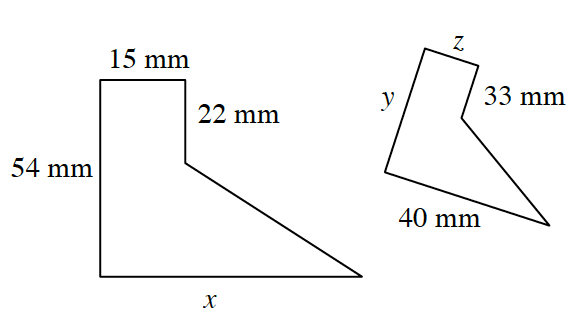### Home > CC3 > Chapter 8 > Lesson 8.1.1 > Problem8-12

8-12.

Find the lengths of the missing sides on the similar shapes at right.Similar shapes have sides with equal ratios to each other. To find each unknown side, compare its ratio to a known side.

For example, the ratio of the $15$ mm side to the $22$ mm side should be equal to the ratio of the z side to the
$33$ mm side.

$\frac{15}{22}=\frac{\it z}{33}$

$z = 22.5 \text{ mm}$

You can now use the value of $z$ to find ratios for the other unknown sides. Write two more ratios to solve for side $x$ and side $y$.

$\frac{15}{54}=\frac{22.5}{\it y}$

$15y = \left(22.5\right) \left(54\right)$

$y = 81 \text{ mm}$
What does $x$ equal?